## What is Multivariate Sensitivity Study?

Multivariate Sensitivity Study calculates the multidimensional solution space (hypercube) of a CHEMCAD or a DWSIM flowsheet simulation. The number of input parameters can be increased freely to widen the solution space.

For a fast calculation, Multivariate Sensitivity Studies are calculated on a cluster of CHEMCAD / DWSIM instances.

It is for every engineer to visualize solution spaces and pareto fronts for multi-objective optimization problems to support decision making.

## Why use the Multivariate Sensitivity Study?

### Cover large solution spaces

You analyze the entire solution space with full factorial or space-filling designs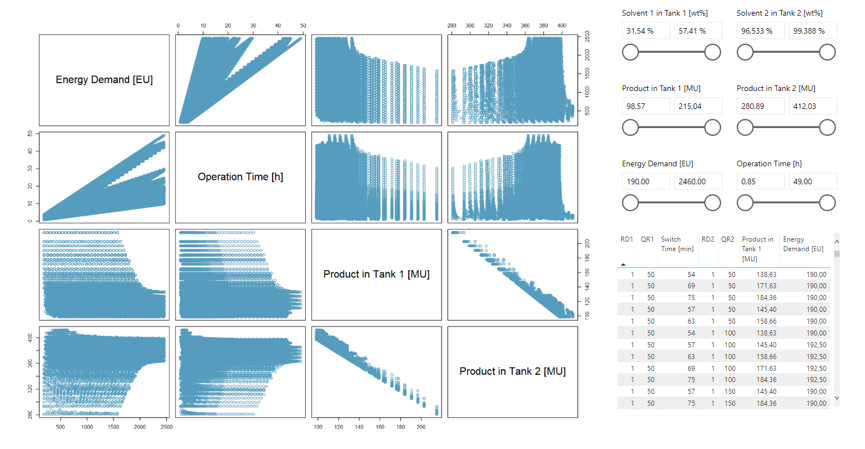### Apply constraints with filters

You discover parameter dependencies and dimension reductions using big data analytics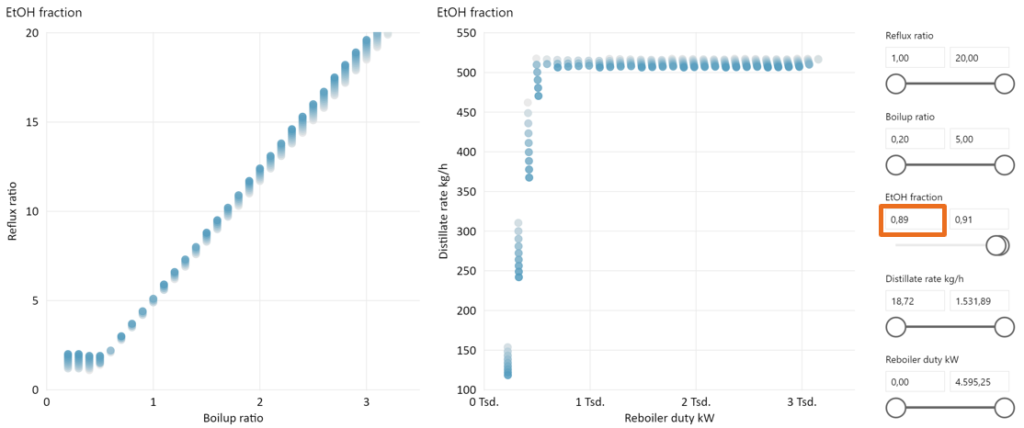### Find pareto-optimal solutions

You visualize solution spaces and pareto fronts for multi-objective optimization problems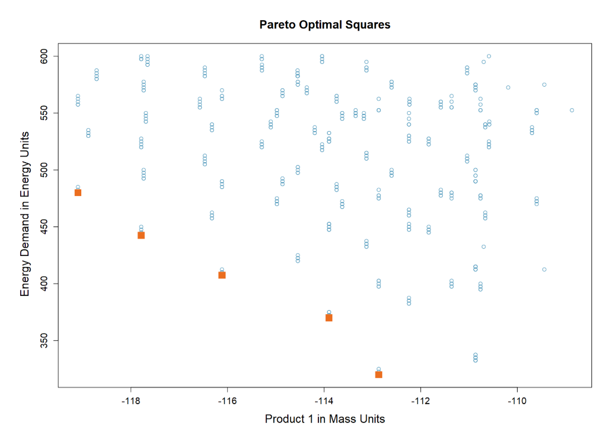## Challenges

• Minimize energy demand
• Minimize operation time
• Maximize product yield
• Product concentration as a constraint
• Five design variables
• Equidistant grid (per variable)
• 78.125 flowsheet calculated (mass transfer batch column simulation)

Increased the solvent recovery efficiency

• 74% energy saving
• 4.5 h reduction in operation time
• 7% increase in product yield
• Infeasible design specifications cause convergence problems
• Due to product accumulation, product specifications must be given indirectly as design specifications
• The correlation of design and state variables are not obvious

## Results

• Maximize product yield
• Minimize energy demand
• Product concentration as a constraint

• Two design variables
• Equidistant grid (per variable)
• 9.550 flowsheet calculated (SCDS)

Understand the correlations between variables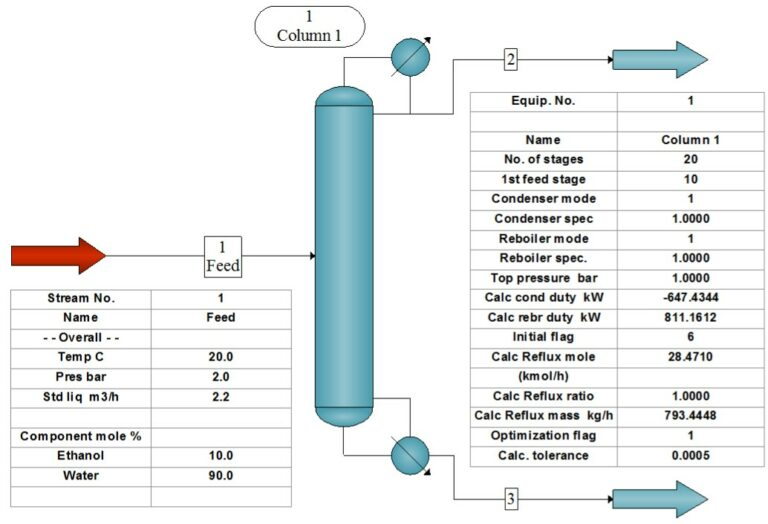## Flowsheet (DWSIM)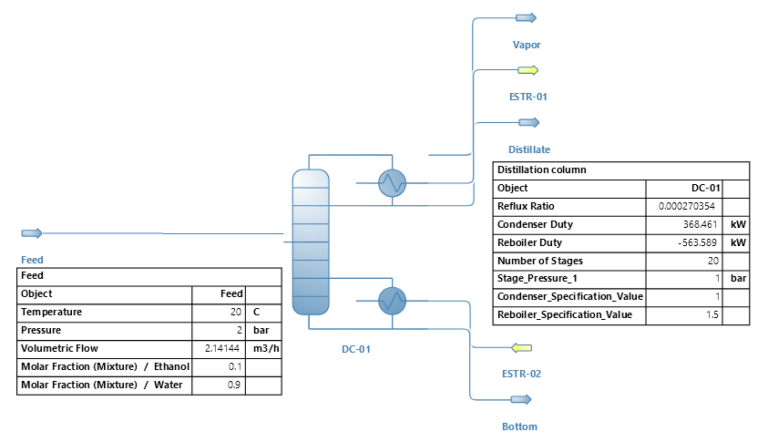## Interactive Data Explorer

Use the sliders on the report below to apply limitations! Observe the relationship between variables.

Check the sensitivities by varying:
• EtOH fraction
• Distillate rate
• Reboiler duty